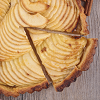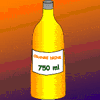#### You may also like### Pumpkin Pie Problem

Peter wanted to make two pies for a party. His mother had a recipe for him to use. However, she always made 80 pies at a time. Did Peter have enough ingredients to make two pumpkin pies?### The Tree and the Greenhouse

Mum, Dad, Tim and Jill moved into a new house. If they cut down the big tree, will it miss the greenhouse?### Orange Drink

A 750 ml bottle of concentrated orange squash is enough to make fifteen 250 ml glasses of diluted orange drink. How much water is needed to make 10 litres of this drink?

# Cherry Buns

##### Age 7 to 11 Challenge Level:

There were several different ways to approach this problem. We received many solutions from children at Ardingly College who all tackled it in a similar way. Here is Jess' reasoning:

I wrote out the ingredients of the cherry buns and showed it like this:

egg = $2$
flour = $2$ eggs
sugar = $2$ eggs
butter = $2$ eggs
cherries = $1$ egg
total = $9$ eggs

First I did $12 \times 45$g = $540$g (the total weight of the mixture)
$540/9$ = $60$g so one egg weighs $60$g.

Alistair from Histon Junior School wrote Jess' solution in a slightly shorter way:

If e = $1$ egg, there are $9$e in the recipe.
I multiplied $45$ by $12$ to get the total weight of mixture. $45x12 =540$
So an egg would be $540/9$ which is $60$, (then turn it into grams) making e = $60$g

Pupils from Oakwood Junior School did it a slightly different way. This is what Sophie wrote:

First I found out how much mixture there was by multiplying $45$g by $12$ paper cases. This gave me an answer of $540$g.
Then next I worked out how much of each ingredient there was in each case.
Eggs $10$g
Flour $10$g
Sugar $10$g
Butter $10$g
Cherries $5$g
Then I worked out how much mixture there was altogether for the 12 cakes:
Eggs $120$g
Flour $120$g
Sugar $120$g
Butter $120$g
Cherries $60$g
After this I halved the amount for the eggs and this gave me $60$g for one egg.

Davis from Berkeley Preparatory School used a trial and improvement approach:

First, my teacher and I found out how many grams the batter weighed by multiplying $45$grams times $12$ paper cake cases.
That means the total batter weighed $540$ grams.
Then, we wrote a formula:
Eggs + flour + sugar + butter + cherries = $540$ grams.
Since the eggs, flour, sugar, and butter all weighed exactly the same, at first we guessed that each ingredient weighed $100$ grams.
That would mean $100$g + $100$g + $100$g + $100$g + cherries (which weigh as much as half of the eggs...which would be $50$g)
However, when we added that together, it only equalled $450$g.
That told me that each ingredient had to weigh more than $100$ grams. So I decided to try $120$ grams.
$120$g + $120$g + $120$g + $120$g + cherries ($60$g) = $540$grams
Now that I know that TWO eggs equals the same as $120$g, ONE egg would equal $60$ grams.

Thank you Davis. Beth, Jennie and Henry found another way to answer the problem:

We set about solving it like this:
She put $45$g in each of $12$ cake cases. That is $12 \times 45$g = $540$g.
So the total mixture weighs $540$g.
Then we listed the ingredients:
$2$ eggs
flour
sugar
butter
glace cherries
The first $4$ weigh the same but the last one weighs only half.
So we need $540/4.5$. This is the weight of each of the first four ingredients.
$540/4.5$ = $120$ (we found this out by trial and improvement)
So $2$ eggs weigh $120$g and the weight of one egg is $60$g.

We checked our solution by writing out the ingredient list again with the weights and checking that the total was $540$g.

Thank you to everyone.